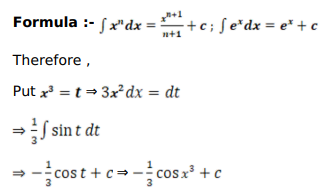# Mark against the correct answer in each of the following:

Question:

Mark $(\sqrt{)}$ against the correct answer in each of the following:

$\int x^{2} \sin x^{3} d x=?$

A. $\cos x^{3}+C$

B. $-\cos x^{3}+C$

C. $-\frac{1}{3} \cos x^{3}+C$

D. none of these

Solution: